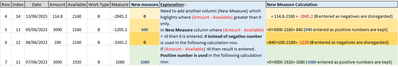cancel
Showing results for
Did you mean:Helper II

## Measure to Deal with Negatives in Calculation

In a previous post:-

https://community.fabric.microsoft.com/t5/Desktop/Use-Previous-Value-In-Column-In-Calculation/m-p/32...

I was kindly supplied with a measure to do the first calculation I need.

Measure =
var _a = ALLSELECTED('Table'[Work Type])
var _b = MAX('Table'[Row])
return SUMX(FILTER(ALL('Table'),[Work Type] in _a&&[Row]<=_b),'Table'[Amount]-'Table'[Available])

Giving the results in the example below
row 1 Measure is Amount - Available  (12 - 2160 = -2148.00)
row 2 Measure is Row 1 Measure (-2148) + Row 2 Amount (85) - Row 2 Available (2160) = -4223
row 3 Measure is Row 2 Measure (-4223) + Row 3 Amount (1660) - Row 3 Available (2106) = -4723I need an additional column which deals diffrently with negatives in the measure column. Therefore the 'New Measure' column instead of -negative numbers uses a 0 and only uses positive numbers in the calculation. See illustration below.I hope this makes sense.

Thanks

Pete

1 ACCEPTED SOLUTIONSuper User

That's unfortunately exactly the point. The result cannot be modified by filters.

4 REPLIES 4Super User

That selective addition is not something you can do in DAX.  You can use List.Accumulate in Power Query for this but that will result in a column, not a measure.Helper II

Thanks @lbendlin . Not sure what list.Accumaulate is, will have a look. I'm not sure it matters if the result is a column so long as it does the calcultion and can be filtered by a slicer.Super User

That's unfortunately exactly the point. The result cannot be modified by filters.Helper II

Thanks for letting me know. I'll have to think of another way of doing it.